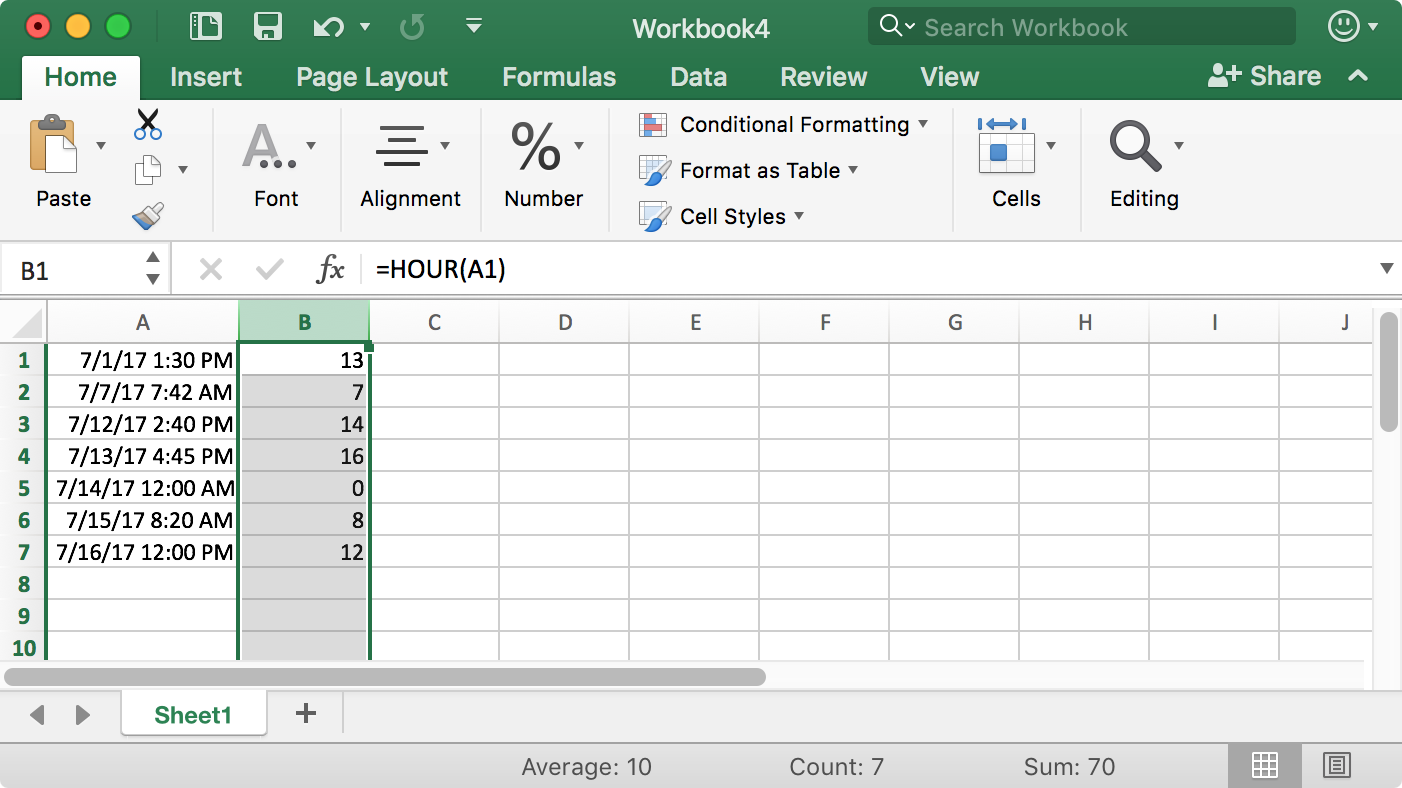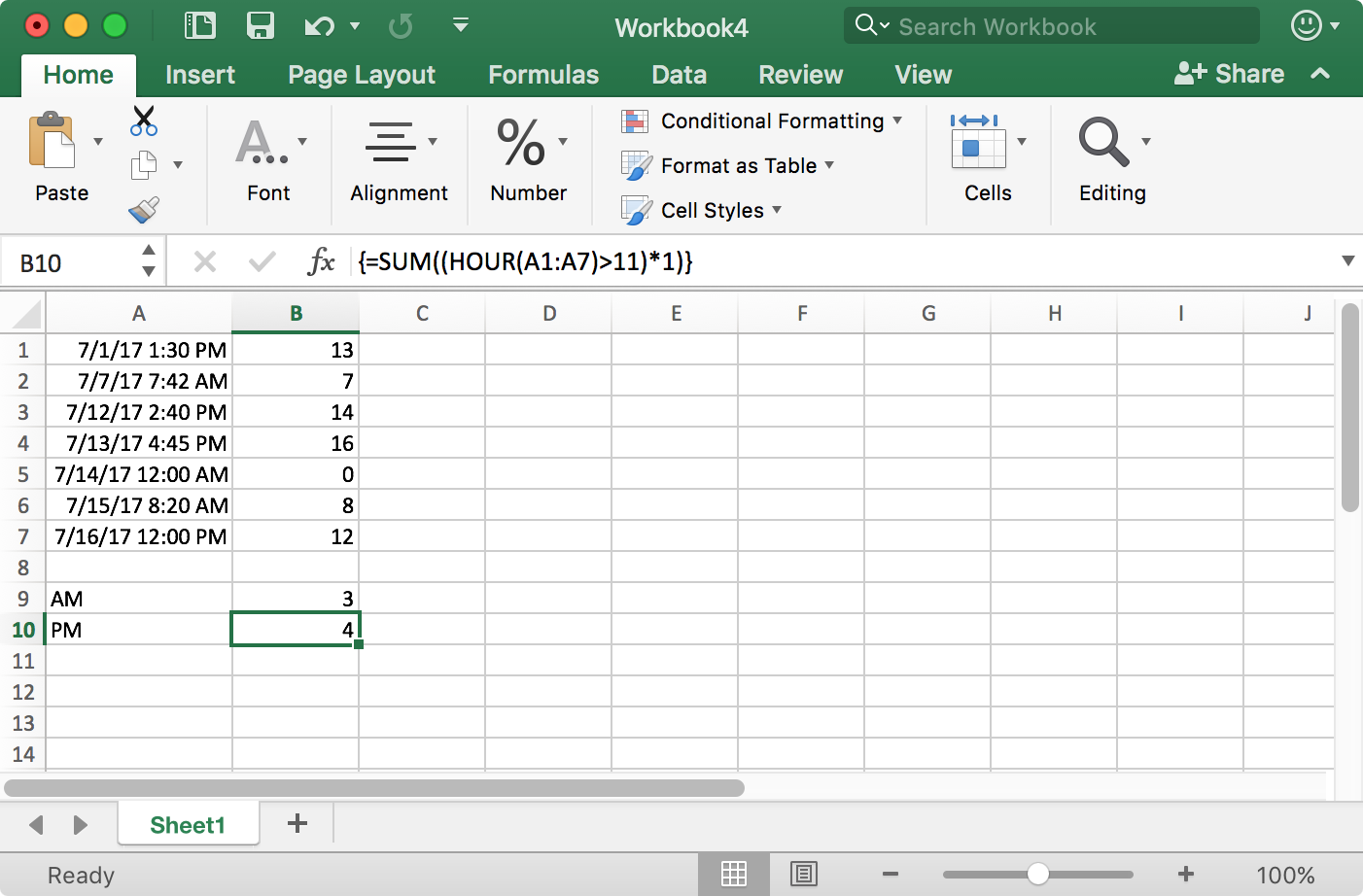# Counting entries in an Excel spreadsheet by AM or PM

Microsoft Excel supports formatting timestamps in a workbook as a date followed by a time with an AM or PM value, i.e., the times are in 12-hour clock format where noon is 12:00 PM and midnight is 12:00 AM. You can select that format for a cell or column in a worksheet by highlighting the cell(s) or column (a column can be selected by clicking on the letter at the top of the column) and then clicking on Format then Cells and then clicking on Date and selecting a type of "3/14/15 1:30 PM."

If you wanted to extract just the hour from the timestamp, you can use the `HOUR` function, e.g. `=HOUR(A1)` to extract the hour value from cell A1. The hour will be displayed in 24-hour clock format, aka military time, i.e., 7:00 AM is 7 while 7:00 PM is 12 plus 7, i.e., 19; 12:00 AM (midnight) is 0 and 12:00 PM (noon) is 12. For instance, give timestamps in column A with the time displayed as AM or PM values and using the HOUR function in a formula for the cells in column B, I would see the following:formulas quick and easyA B 1 7/1/17 1:30 PM 13 2 7/7/17 7:42 AM 7 3 7/12/17 2:40 PM 14 4 7/13/17 4:45 PM 16 5 7/14/17 12:00 AM 0 6 7/15/17 8:20 AM 8 7 7/16/17 12:00 PM 12To count the number of cells that have an AM value and the number of cells that have a PM value, you can use an array formula. Again, assuming that column A holds the time stamps and as in the example above, cells A1 through A7 contain the timestamp entries, I could use the following formulas:

 AM =SUM((HOUR(A1:A7)<12)*1) PM =SUM((HOUR(A1:A7)>11)*1)

Note: you have to hit control-shift-enter, i.e., hit the control, shift, and enter keys simultanesouly, after typing the formulas to make them array formulas. When you do so, you will see the formulas get curly brackets, aka braces, around them as shown below:

 AM {=SUM((HOUR(A1:A7)<12)*1)} PM {=SUM((HOUR(A1:A7)>11)*1)}

For the example above, you would see the following:Example workbook: Count_By_AM_PM.xlsx

References: